WHCSRL 技术网

# 游戏引擎学习记录（2）- 渲染（OpenGL）- 变换操作

## 矩阵（Matrix）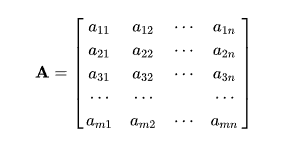通常情况下在点坐标的表示时，会用矩阵保存点坐标。在这里先不做赘述了。

## 齐次坐标

1. 常见的三种变换操作：旋转、平移和缩放，平移在表示的时候通常是以矩阵加减法的形式来计算，但旋转和缩放通常是用矩阵乘除法。这样的话在计算上以及代码书写上会增加计算量并在书写上造成麻烦。引入齐次坐标的目的主要是合并矩阵运算中的乘法和加法，它提供了用矩阵运算把二维、三维甚至高维空间中的一个点集从一个坐标系变换到另一个坐标系的有效方法。因此在矩阵变换上，三种矩阵最后连乘即可得到的图像矩阵。

2. 能够简化透视投影的计算，这部分我们后面再谈。

## 空间变换

OpenGL的空间变换包括以下三种：

Model matrix 模型矩阵。进行物体坐标系到世界坐标系的转换。控制了物体的平移、旋转、缩放。在3D建模软件中为模型坐标，导入游戏后使用model martrix进行大小、位置、角度的相关设置。
View matrix 观察矩阵。将世界坐标系变换到观察者坐标系，通过一些平移、旋转的组合来移动整个场景（而不是去移动摄像机，摄像机是一个虚拟的概念，事实上代码中并没有摄像机camera， 而是用view martix来表示摄像机，然后把view matrix附加到每一个物体，来模拟相关的摄像机操作），用来模拟一个摄像机。
projection matrix 投影矩阵。将观察者坐标系转换到裁剪坐标系。将3D坐标投影到2D屏幕上，裁剪空间外的顶点会被裁掉，投影矩阵指定了坐标的范围。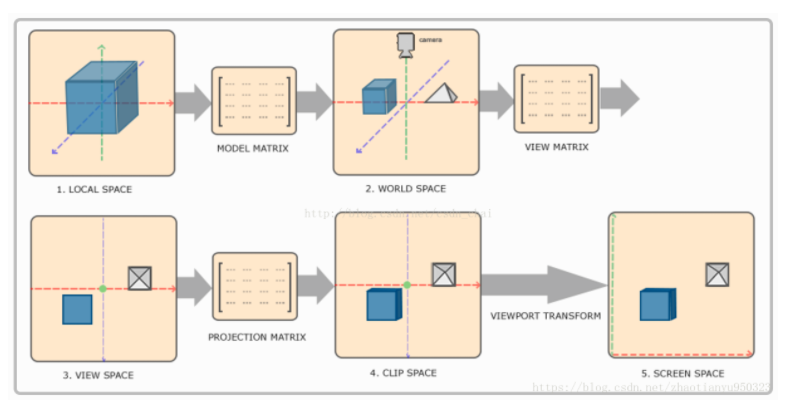## 正交投影以及透视投影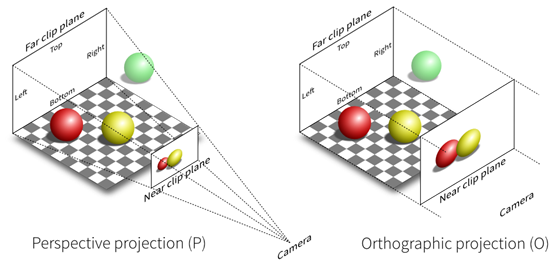glMatrixMode(GL_PROJECTION); //将当前矩阵指定为投影矩阵

## 实现

``#pragma once#include "../nclgl/OGLRenderer.h"class Renderer :public OGLRenderer {public:	Renderer(Window& parent);	virtual ~Renderer(void);	virtual void RenderScene(); 	//今天要实现的函数：正交投影 透视投影 缩放、旋转、平移	void SwitchToPerspective();	void SwitchToOrthographic();	inline void SetScale(float s) { scale = s; }	inline void SetRotation(float r) { rotation = r; }	inline void SetPosition(Vector3 p) { position = p; } protected:	Mesh* triangle;	Shader* matrixShader;	float scale;	float rotation;	Vector3 position;};``

``void Renderer::SwitchToOrthographic(){	projMatrix = Matrix4::Orthographic(-1.0f, 10000.0f, width / 2.0f, -width / 2.0f, height / 2.0f, -height / 2.0f);}void Renderer::SwitchToPerspective(){	projMatrix = Matrix4::Perspective(1.0f, 10000.0f, (float)width / (float)height, 45.0f);}``

`` Matrix4 Matrix4::Perspective(float znear, float zfar, float aspect, float fov) {	Matrix4 m; 	const float h = 1.0f / tan(fov*PI_OVER_360);	float neg_depth = znear-zfar; 	m.values		= h / aspect;	m.values		= h;	m.values	= (zfar + znear)/neg_depth;	m.values	= -1.0f;	m.values	= 2.0f*(znear*zfar)/neg_depth;	m.values	= 0.0f; 	return m;} //http://www.opengl.org/sdk/docs/man/xhtml/glOrtho.xmlMatrix4 Matrix4::Orthographic(float znear, float zfar,float right, float left, float top, float bottom)	{	Matrix4 m; 	m.values	= 2.0f / (right-left);	m.values	= 2.0f / (top-bottom);	m.values	= -2.0f / (zfar-znear); 	m.values  = -(right+left)/(right-left);	m.values  = -(top+bottom)/(top-bottom);	m.values  = -(zfar+znear)/(zfar-znear);	m.values  = 1.0f; 	return m;}``

void glOrtho(GLdouble left, GLdouble right, GLdouble bottom, GLdouble top, GLdouble zNear, GLdouble zFar);

void gluOrtho2D(GLdouble left, GLdouble right, GLdouble bottom, GLdouble top);

void glFrustum(GLdouble left, GLdouble right, GLdouble bottom, GLdouble top, GLdouble zNear, GLdouble zFar);

void gluPerspective(GLdouble fovy, GLdouble aspect, GLdouble zNear, GLdouble zFar);

void glFrustum(GLdouble left, GLdouble Right, GLdouble bottom, GLdouble top, GLdouble near, GLdouble far);

left,right       表示近平面左右两边相对于垂直平面的位置

bottom,top   表示近平面顶和底相对于水平面的位置

zNear和zFar是表示视点到远近投影平面的距离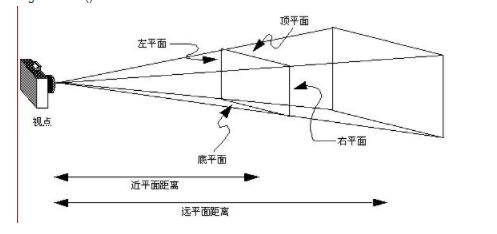gluPerspective(GLdouble fovy,GLdouble aspect,GLdouble zNear,GLdouble zFar);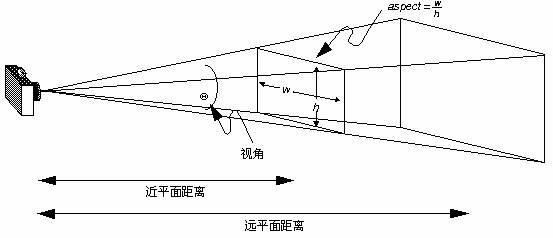理解：glFrustum 和 gluPerspective都是透视矩阵的操作，后者是对前者的简单封装，后者不再需要上下左右的位置，需要fovy和aspect，fovy是视角大小，aspect是视景体的宽高比（观点有误请指出，不胜感激）

下一步我们实现RenderScene函数：

``void Renderer::RenderScene(){	glClear(GL_COLOR_BUFFER_BIT);	BindShader(matrixShader);	glUniformMatrix4fv(glGetUniformLocation(matrixShader->GetProgram(), "projMatrix"), 1, false, projMatrix.values);	glUniformMatrix4fv(glGetUniformLocation(matrixShader->GetProgram(), "viewMatrix"), 1, false, viewMatrix.values); 	for (int i = 0; i < 3; ++i)	{		Vector3 tempPos = position;		tempPos.z += (i * 500.0f);		tempPos.x -= (i * 100.0f);		tempPos.y -= (i * 100.0f); 		modelMatrix = Matrix4::Translation(tempPos) * Matrix4::Rotation(rotation, Vector3(0, 1, 0)) * Matrix4::Scale(Vector3(scale, scale, scale));		glUniformMatrix4fv(glGetUniformLocation(matrixShader->GetProgram(),"modelMatrix"), 1, false, modelMatrix.values);		triangle->Draw();	}}``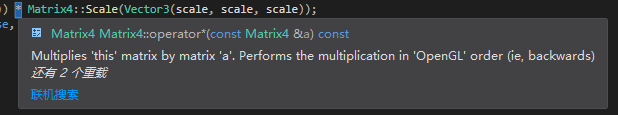这里发现矩阵乘法的重载是遵循OpenGL的顺序，也就是先乘（从右向左），看重载实现：

``	//Multiplies 'this' matrix by matrix 'a'. Performs the multiplication in 'OpenGL' order (ie, backwards)	inline Matrix4 operator*(const Matrix4 &a) const{			Matrix4 out;		//Students! You should be able to think up a really easy way of speeding this up...		for(unsigned int r = 0; r < 4; ++r) {			for(unsigned int c = 0; c < 4; ++c) {				out.values[c + (r*4)] = 0.0f;				for(unsigned int i = 0; i < 4; ++i) {					out.values[c + (r*4)] += this->values[c+(i*4)] * a.values[(r*4)+i];				}			}		}		return out;	}``

``#version 330 coreuniform mat4 modelMatrix;uniform mat4 viewMatrix;uniform mat4 projMatrix; in vec3 position;in vec4 colour; out Vertex{	vec4 colour;} OUT; void main(void){	mat4 mvp = projMatrix*viewMatrix*modelMatrix;	gl_Position = mvp*vec4(position,1.0);	OUT.colour = colour;}``

oid glUniformMatrix4fv (GLint location, GLsizei count, GLboolean transpose, const GLfloat * value)

location : uniform的位置。
count : 需要加载数据的数组元素的数量或者需要修改的矩阵的数量。
transpose : 指明矩阵是列优先(column major)矩阵（GL_FALSE）还是行优先(row major)矩阵（GL_TRUE）。
value : 指向由count个元素的数组的指针。

m00 m01

m10 m11

m00 m10

m01 m11

Renderer.cpp完整如下：

``#include "Renderer.h" Renderer::Renderer(Window& parent) : OGLRenderer(parent){	triangle = Mesh::GenerateTriangle();	//颜色的片段着色器不动 需要写一个新的矩阵顶点shader	matrixShader = new Shader("MatrixVertex.glsl", "colourFragment.glsl");	if (!matrixShader->LoadSuccess())	{		return;	}	init = true;	SwitchToOrthographic();//切换到正交投影}Renderer::~Renderer(void){	delete triangle;	delete matrixShader;}void Renderer::SwitchToOrthographic(){	projMatrix = Matrix4::Orthographic(-1.0f, 10000.0f, width / 2.0f, -width / 2.0f, height / 2.0f, -height / 2.0f);}void Renderer::SwitchToPerspective(){	projMatrix = Matrix4::Perspective(1.0f, 10000.0f, (float)width / (float)height, 45.0f);}void Renderer::RenderScene(){	glClear(GL_COLOR_BUFFER_BIT);	BindShader(matrixShader);	glUniformMatrix4fv(glGetUniformLocation(matrixShader->GetProgram(), "projMatrix"), 1, false, projMatrix.values);	glUniformMatrix4fv(glGetUniformLocation(matrixShader->GetProgram(), "viewMatrix"), 1, false, viewMatrix.values); 	for (int i = 0; i < 3; ++i)	{		Vector3 tempPos = position;		tempPos.z += (i * 500.0f);		tempPos.x -= (i * 100.0f);		tempPos.y -= (i * 100.0f); 		modelMatrix = Matrix4::Translation(tempPos) * Matrix4::Rotation(rotation, Vector3(0, 1, 0)) * Matrix4::Scale(Vector3(scale, scale, scale));		glUniformMatrix4fv(glGetUniformLocation(matrixShader->GetProgram(),"modelMatrix"), 1, false, modelMatrix.values);		triangle->Draw();	}}``

``#include "../nclgl/window.h"#include "Renderer.h" int main() {	Window w("Vertex Transformation!", 800, 600, false);	if (!w.HasInitialised()) {		return -1;	} 	Renderer renderer(w);	if (!renderer.HasInitialised()) {		return -1;	} 	float scale = 100.0f;	float rotation = 0.0f;	Vector3 position(0, 0, -1500.0f); 	while (w.UpdateWindow() && !Window::GetKeyboard()->KeyDown(KEYBOARD_ESCAPE)) {		if (Window::GetKeyboard()->KeyDown(KEYBOARD_1))			renderer.SwitchToOrthographic();		if (Window::GetKeyboard()->KeyDown(KEYBOARD_2))			renderer.SwitchToPerspective(); 		if (Window::GetKeyboard()->KeyDown(KEYBOARD_PLUS))  ++scale;		if (Window::GetKeyboard()->KeyDown(KEYBOARD_MINUS)) --scale; 		if (Window::GetKeyboard()->KeyDown(KEYBOARD_LEFT))  ++rotation;		if (Window::GetKeyboard()->KeyDown(KEYBOARD_RIGHT)) --rotation; 		if (Window::GetKeyboard()->KeyDown(KEYBOARD_K))			position.y -= 1.0f;		if (Window::GetKeyboard()->KeyDown(KEYBOARD_I))			position.y += 1.0f; 		if (Window::GetKeyboard()->KeyDown(KEYBOARD_J))			position.x -= 1.0f;		if (Window::GetKeyboard()->KeyDown(KEYBOARD_L))			position.x += 1.0f; 		if (Window::GetKeyboard()->KeyDown(KEYBOARD_O))			position.z -= 1.0f;		if (Window::GetKeyboard()->KeyDown(KEYBOARD_P))			position.z += 1.0f; 		renderer.SetRotation(rotation);		renderer.SetScale(scale);		renderer.SetPosition(position);		renderer.RenderScene();		renderer.SwapBuffers();	} 	return 0;}``

## 结果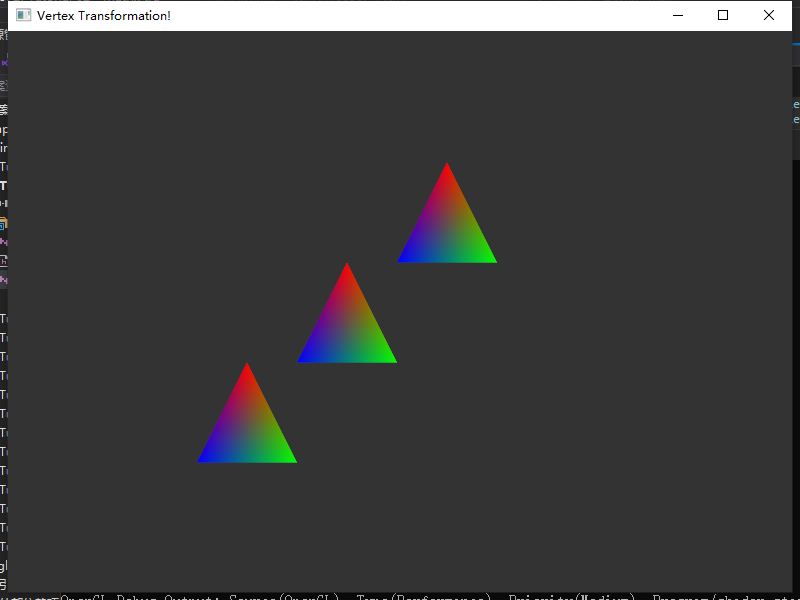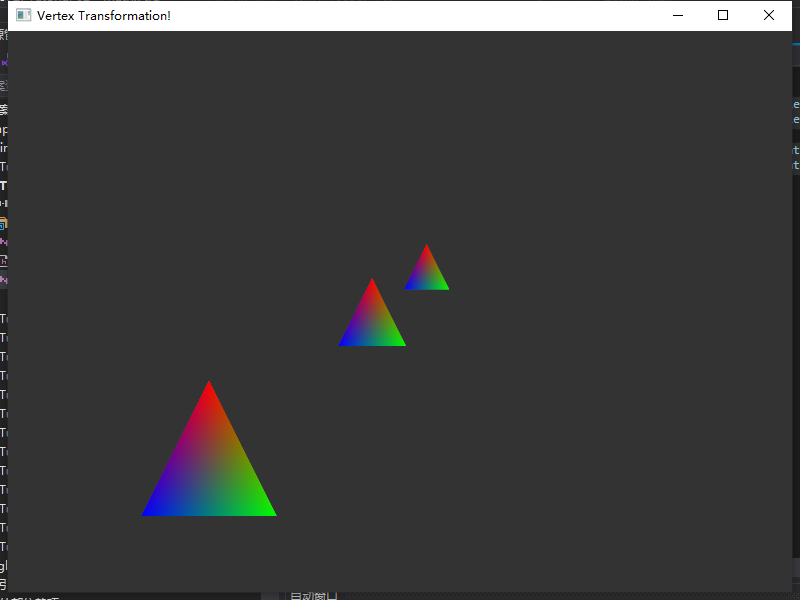自此，我们已经完成空间变换的操作，并且可以通过键盘按键控制他的平移及旋转。